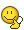## Recommended Posts

Hi,

We just started chemestry at school and I'm really not getting it very well...

Here is an experiment we did in lab that I don't fully understand (the purpose..): we got this sample of a this contaminated river and it had pH of 9.

I took 5ml of it then added 0.16ml of HCL (at 1mol/ml), and it now gives us a pH of 7...

Is there a way to get the concentration of the first liquid out of this? I really don't understand this stuff....

##### Share on other sites

Well, the water was neutralized with the HCl solution that contributed 0.16mol of hydrogen ions, so there had to be 0.16mol of hydroxide ions in the 5ml; a 0,032mol/ml concentration. I'm not really sure what use that is though, as you could've derived the concentration from the original pH itself... or is that what they are trying to teach you at the moment? I guess that serves as an adequate demonstration of the logarithmic nature of the pH scale then.

##### Share on other sites

I don't know...It's really messed up...

Is 0.16mol the necessary quantity to neutralize the liquid we started with (which is NaOH added to some water)?

Is there a way for me to find out the concentration from what I have? (I don't understand any logic in all this...if anyone can explain it it would be great.)

And Gilded, how did you get the 0.032?

Edited by hitmankratos

##### Share on other sites
I don't know...It's really messed up...

Is 0.16mol the necessary quantity to neutralize the liquid we started with (which is NaOH added to some water)?

Is there a way for me to find out the concentration from what I have? (I don't understand any logic in all this...if anyone can explain it it would be great.)

And Gilded, how did you get the 0.032?

Yes 0.16mol is the necessary amount. Since NaOH is a mono... something base (can't remember the English term) and contributes just one -OH, equally many molecules of HCl are needed. Thus the NaOH concentration is the 0.032mol/ml I mentioned. 0.16mol/5ml (original amount of liquid) = 0.032mol/ml.

I hope I've gotten this right so far, it's been quite some time since I've done even these kind of simple calculations.

##### Share on other sites

lol, simple calculations !

Sorry if the following is going to seem really dumb, but what does 0.032mol/ml represent? What does it mean? It's all numbers and facts but I just don't understand the point of doing this stuff...

##### Share on other sites

Well obviously it represents pH and alkalinity.Also, you can figure out the mass of the NaOH that's in there for example, just like you could from any molar quantity, and with calculations like these you can also figure out what sort of solution you need to have to achieve a certain pH. But in the end it's just basic (pun sort of intended) practice I guess.##### Share on other sites
Well obviously it represents pH and alkalinity.

What??

I don't get it(Sorry). Does it mean it has 0.032mol/L of pH ??? Is that what you're saying?:confused:##### Share on other sites

Hello,

What you are describing is a common "titration" problem, wherebye you use a recorded volume of an acid at a known concentration (this is called a "standardized solution") to measure the concentration of an unknown base.

A "pH" value gives you an idea of the concentration of hydrogen ions in a solution. The scale runs from 1-14 and increases with basicity. So 1 is very highly acidic, 14 is highly basic, and 7 describes a neutral substance.

the pH 9 value tells you that you have a basic solution. When you add a strong acid (like HCL) to a basic solution it will lower the pH as it neutralizes the base. The equation is (since the base is uknown I'll just refer to it as X, followed by 'OH' to indicate basicity):

HCL + XOH ---> XCL + H20

Acids and bases come together to form water. Next, you know that the HCL you used is of the concentration 1 mol / mL. This is refered to as "molarity", and the equation is given by:

Molarity = moles / volume

First convert mL to L (0.16 mL = .00016 L), 1 = x/(.00016), (so x, or "number of moles", is .00016). Since it took x moles of acid to "neutralize" the alkilinity to a pH of 7 (which is nuetral) you know that you have "used up" all of the present base. Therefore the amount # of moles of base is is equal to the # moles of acid.

Now that you know how many moles of base there were, you can use the molarity equation to solve for concentration base. Use the volume of the "polluted water" that you picked up, which was 5ml (= .005 L)

So Molarity base = (# moles base)/(volume base) = (.00016 mole / .005L ) = 0.032 n / L

## Create an account

Register a new account